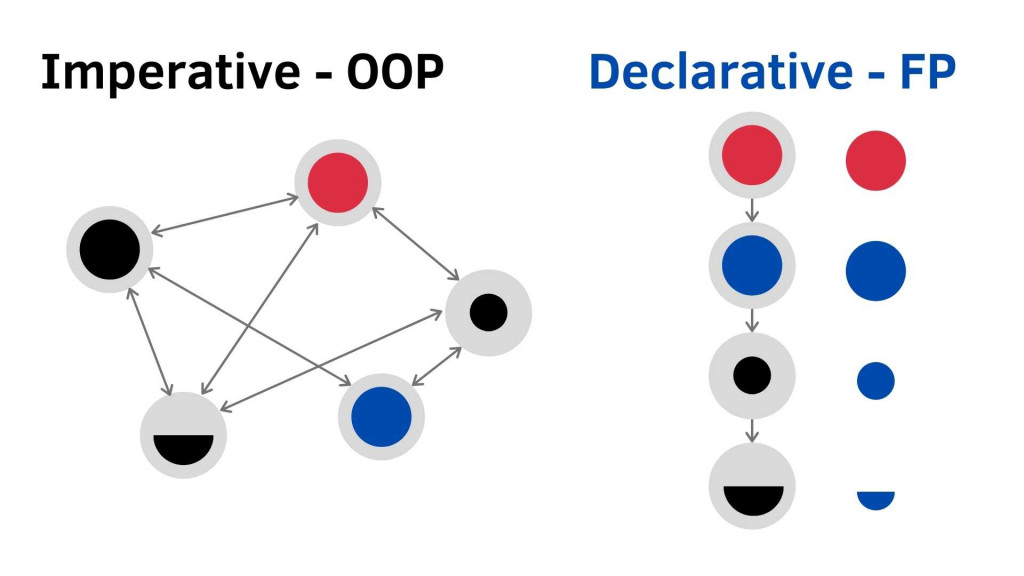#DAY 8
1
Software Development

## Buzz word 4 : Stateless

Stateless is any variable, object, or memory space that exists in a shared scope, or as the property of an object being passed between scopes.

shared scope 可以包含在 global scope 或是 function scope (恩，其實 JS 總共也才這兩個 scope)。

``````// words = ['world', 'fp', 'to', 'welcome']
let keepers = []

for(let i in words) {
if(words[i].length > 3) {
keepers.push(words[i])
}  // ['world', 'welcome']
}
// 程式大家一起 Share keepers 這個 Array
``````

Shared state 最大問題就是你必須很清楚每一行程式碼在做什麼，什麼時候做了什麼事變動了 state，並且要記憶 state 變成多少 。一但系統變複雜或是有多個工程師共同開發就必須花大量時間去弄懂程式碼在幹嘛 (崩潰狀)

``````// Stateful
const x = 4;
x++; // x 變 5
// 省略 100 行...

x*2 // 完全不知道哪行改過 x，現在到底變成什麼

````````````// Stateless
_.filter(x => x.length > 3),  // 不用記憶 x 是什麼
``````

``````const x = {
val: 2
};

const x1 = () => x.val += 1;

const x2 = () => x.val *= 2;

x2();
x1();

console.log(x.val); // 5

x2();
x1();
console.log(x.val); // 11

``````

``````const x = {
val: 2
};

const x1 = x => Object.assign({}, x, { val: x.val + 1});
const x2 = x => Object.assign({}, x, { val: x.val * 2});

console.log(x1(x2(x)).val); // 5

x2(x);
x1(x);
// 那順序改變呢
console.log(x1(x2(x)).val); // 5

``````

#### 參考文章

``````如有錯誤或需要改進的地方，拜託跟我說。

``````